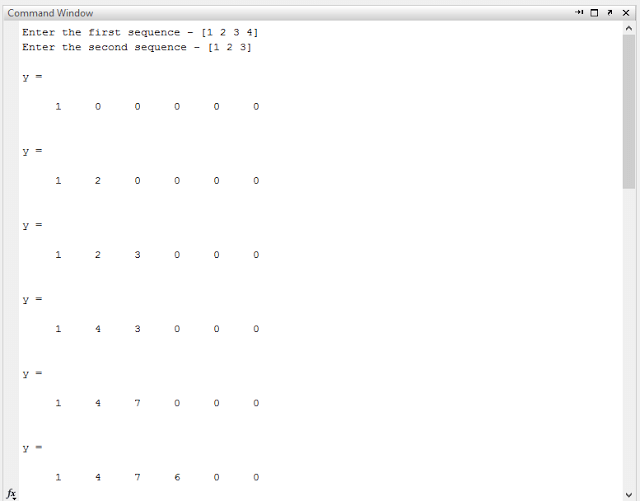# Linear Convolution

## Aim: To perform linear convolution using MATLAB

### Requirements:

MATLAB 2007 and above (another version may also work but I haven’t tried personally)

### Theory

Convolution is a formal mathematical operation, just as multiplication, addition, and integration. Addition takes two numbers and produces a third number, while convolution takes two signals and produces a third signal. Convolution is used in the mathematics of many fields, such as probability and statistics. In linear systems, convolution is used to describe the relationship between three signals of interest: the input signal, the impulse response, and the output signal.

If the input and impulse response of a system are x[n] and h[n] respectively, the convolution is given by the expression,

x[n] * h[n] = ε x[k] h[n-k]

Where k ranges between -∞ and ∞

If,

x(n) is an M- point sequence
h(n) is an N – point sequence
then, y(n) is a (M+N-1) – point sequence.

In this equation, x(k), h(n-k) and y(n) represent the input to and output from the system at time n. Here we could see that one of the inputs is shifted in time by a value every time it is multiplied with the other input signal. Linear Convolution is quite often used as a method of implementing filters of various types.

In mathematics and, in particular, functional analysis, convolution is a mathematical operation on two functions f and g, producing a third function that is typically viewed as a modified version of one of the original functions, giving the area overlap between the two functions as a function of the amount that one of the original functions is translated. Convolution is similar to cross-correlation. It has applications that include probability, statistics, computer vision, natural language processing, image and signal processing, engineering, and differential equations.

The convolution can be defined for functions on groups other than Euclidean space. For example, periodic functions, such as the discrete-time Fourier transform, can be defined on a circle and convolved by periodic convolution. A discrete convolution can be defined for functions on the set of integers. Generalizations of convolution have applications in the field of numerical analysis and numerical linear algebra, and in the design and implementation of finite impulse response filters in signal processing.

Matlab allows us to perform almost all of the digital signal processing applications on software, which makes it a lot easier to study the principles of digital signal processing and digital communication. Matlab almost performs all the operations regarding audio video and signals. One of the most important features of Matlab is that we can plot our results and observe them which is difficult manually.

Computing the inverse of the convolution operation is known as deconvolution.

## Matlab Code for Linear Convolution

### Results

#### Input#### OutputResult: Through this experiment, we were able to verify linear convolution using Matlab, we can verify circular convolution using linear convolution you can check below post

Here are some other programs of DSP Matlab

What is System Programming?

#### Are You in Search of A Job?

Subscribe to get job Alerts straight to your email inbox absolutely Free!

Thank you for subscribing.

Something went wrong.